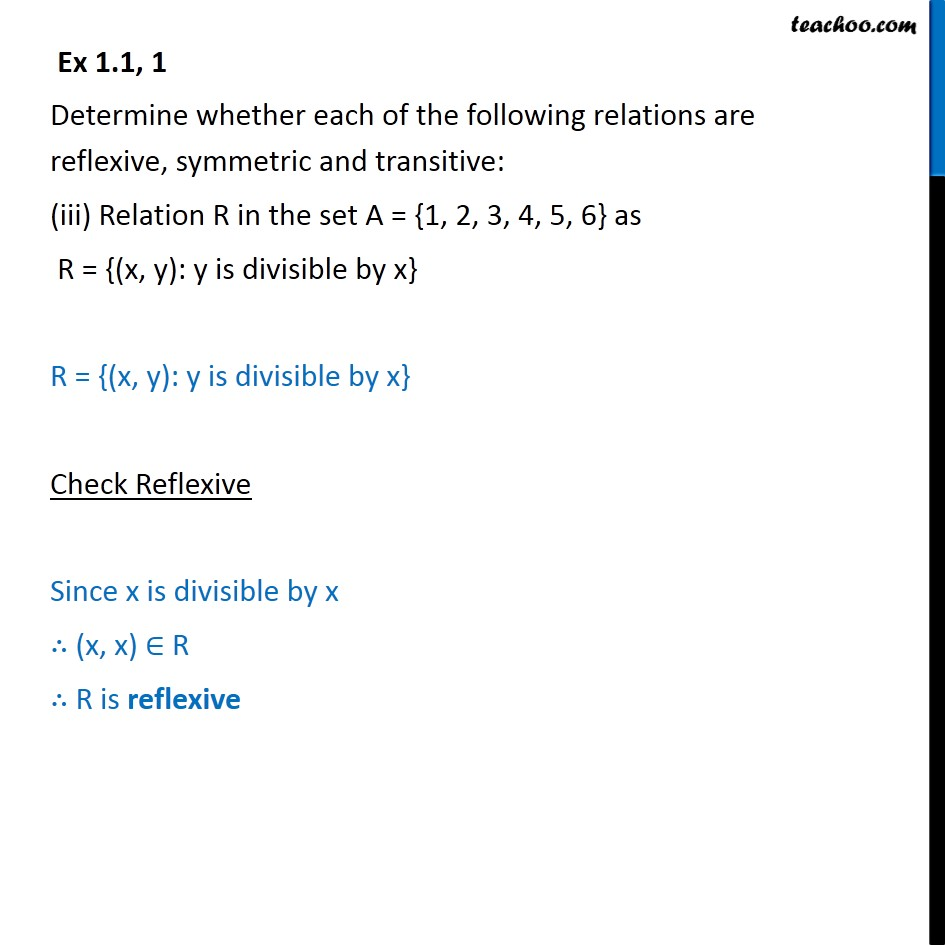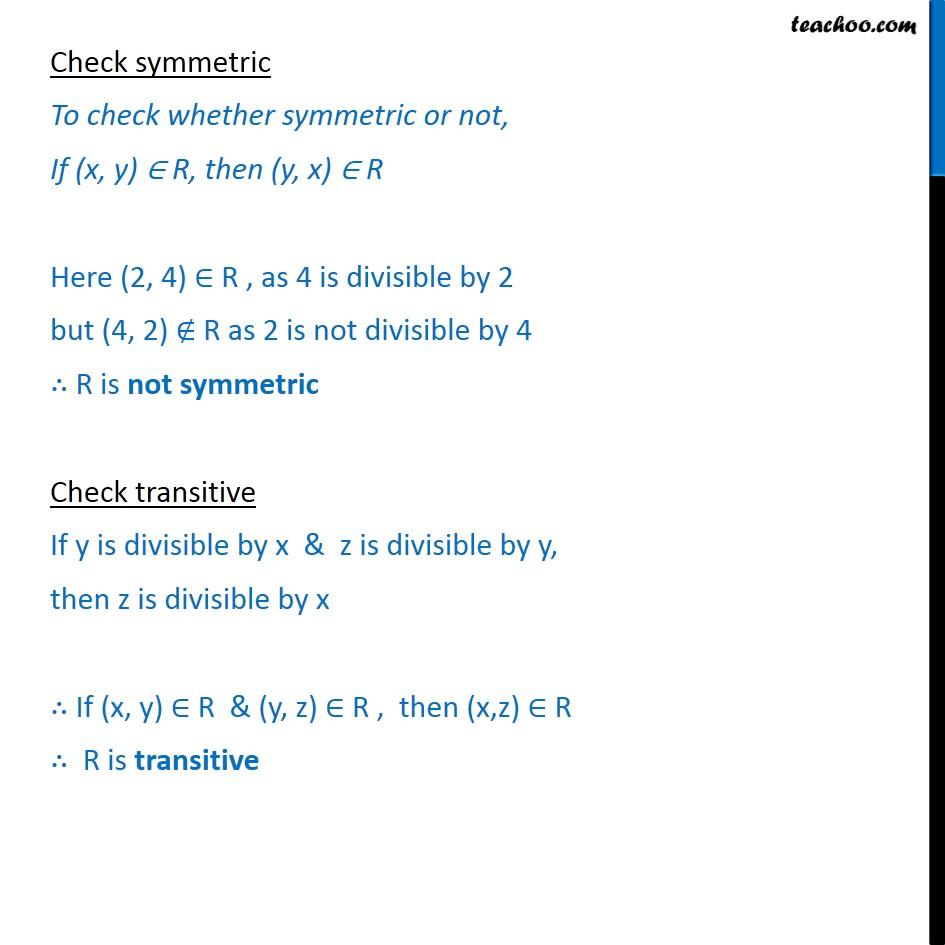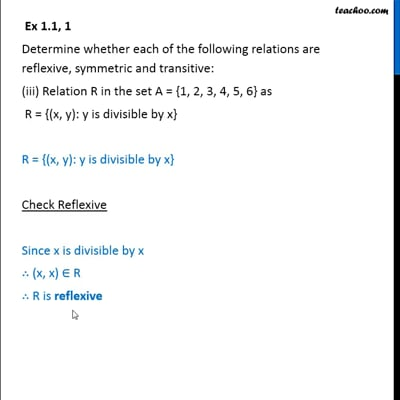Ex 1.1

Chapter 1 Class 12 Relation and Functions
Serial order wiseThis video is only available for Teachoo black users

Maths Crash Course - Live lectures + all videos + Real time Doubt solving!

### Transcript

Ex 1.1, 1 Determine whether each of the following relations are reflexive, symmetric and transitive: (iii) Relation R in the set A = {1, 2, 3, 4, 5, 6} as R = {(x, y): y is divisible by x} R = {(x, y): y is divisible by x} Check Reflexive Since x is divisible by x (x, x) R R is reflexive Check symmetric To check whether symmetric or not, If (x, y) R, then (y, x) R Here (2, 4) R , as 4 is divisible by 2 but (4, 2) R as 2 is not divisible by 4 R is not symmetric Check transitive If y is divisible by x & z is divisible by y, then z is divisible by x If (x, y) R & (y, z) R , then (x,z) R R is transitive